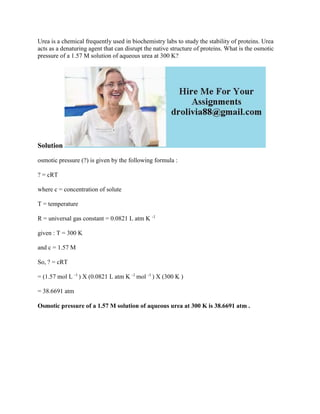Seu SlideShare está sendo baixado. ×

# Urea is a chemical frequently used in biochemistry labs to study the s.docx

Anúncio
Anúncio
Anúncio
Anúncio
Anúncio
Anúncio
Anúncio
Anúncio
Anúncio
Anúncio
AnúncioCarregando em…3
×

1 de 2 Anúncio

# Urea is a chemical frequently used in biochemistry labs to study the s.docx

Urea is a chemical frequently used in biochemistry labs to study the stability of proteins. Urea acts as a denaturing agent that can disrupt the native structure of proteins. What is the osmotic pressure of a 1.57 M solution of aqueous urea at 300 K?
Solution
osmotic pressure (?) is given by the following formula :
? = cRT
where c = concentration of solute
T = temperature
R = universal gas constant = 0.0821 L atm K -1
given : T = 300 K
and c = 1.57 M
So, ? = cRT
= (1.57 mol L -1 ) X (0.0821 L atm K -1 mol -1 ) X (300 K )
= 38.6691 atm
Osmotic pressure of a 1.57 M solution of aqueous urea at 300 K is 38.6691 atm .
.

Urea is a chemical frequently used in biochemistry labs to study the stability of proteins. Urea acts as a denaturing agent that can disrupt the native structure of proteins. What is the osmotic pressure of a 1.57 M solution of aqueous urea at 300 K?
Solution
osmotic pressure (?) is given by the following formula :
? = cRT
where c = concentration of solute
T = temperature
R = universal gas constant = 0.0821 L atm K -1
given : T = 300 K
and c = 1.57 M
So, ? = cRT
= (1.57 mol L -1 ) X (0.0821 L atm K -1 mol -1 ) X (300 K )
= 38.6691 atm
Osmotic pressure of a 1.57 M solution of aqueous urea at 300 K is 38.6691 atm .
.

Anúncio
Anúncio

### Urea is a chemical frequently used in biochemistry labs to study the s.docx

1. 1. Urea is a chemical frequently used in biochemistry labs to study the stability of proteins. Urea acts as a denaturing agent that can disrupt the native structure of proteins. What is the osmotic pressure of a 1.57 M solution of aqueous urea at 300 K? Solution osmotic pressure (?) is given by the following formula : ? = cRT where c = concentration of solute T = temperature R = universal gas constant = 0.0821 L atm K -1 given : T = 300 K and c = 1.57 M So, ? = cRT = (1.57 mol L -1 ) X (0.0821 L atm K -1 mol -1 ) X (300 K ) = 38.6691 atm Osmotic pressure of a 1.57 M solution of aqueous urea at 300 K is 38.6691 atm .